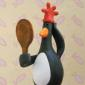# function for stretchy bones

Hi, everyone
I am new in maxscript and I want to create a stretchy leg. I have made a function, it works very well, but when I restart 3dsmax, I have many of errors. I need help, please. Here is the code:

---------------------------

--freeze transform as function
fn FreezeTransformA item =
(
local CurObj = item

if classof CurObj.rotation.controller != Rotation_Layer do
(

-- freeze rotation
CurObj.rotation.controller = Euler_Xyz()
CurObj.rotation.controller = Rotation_list()
CurObj.rotation.controller.available.controller = Euler_xyz()

-- "Localization on" --

CurObj.rotation.controller.setname 1 "Frozen Rotation"
CurObj.rotation.controller.setname 2 "Zero Euler XYZ"

-- "Localization off" --

CurObj.rotation.controller.SetActive 2
)
if classof CurObj.position.controller != Position_Layer do
(

-- freeze position
CurObj.position.controller = Bezier_Position()
CurObj.position.controller = position_list()
CurObj.position.controller.available.controller = Position_XYZ()

-- "Localization on" --

CurObj.position.controller.setname 1 "Frozen Position"
CurObj.position.controller.setname 2 "Zero Pos XYZ"

-- "Localization off" --

CurObj.position.controller.SetActive 2

-- position to zero
CurObj.Position.controller.x_Position = 0
CurObj.Position.controller.y_Position = 0
CurObj.Position.controller.z_Position = 0
)

)
--FreezeTransform \$

select \$*
for i in selection do
(
freezeTransformA i
)

-- Create IK solver

ptA = point box: on name:"ThighUp" cross:off
ptB =point box: on name:"ThighDown" cross:off

\$thighup.pos = \$bone001.pos
\$thighDown.pos = \$bone003.pos
\$bone001.parent = \$thighUp
ab = IKSys.ikChain \$bone001 \$bone003 "IKHISolver"

ab.name = "ikleg"

\$ikleg.transform.controller.goalSize = 10

\$thighDown.parent = \$Ikleg

Function StretchyBonesLegs Bone01 Bone02 Bone03 PointA PointB =
(
-- store lengths of bones
LengthA = Bone01.length
LengthB = Bone02.length

total = LengthA + LengthB

bone02.pos.controller.Zero_Pos_XYZ.controller.X_Position.controller = float_script()
bone02.pos.controller.Zero_Pos_XYZ.controller.X_Position.controller.script =
"total = LengthA + LengthB
dist = (distance pointA pointB)
if dist > total then (
xPos = ((dist - total)/2)
) else (
xPos = 0)"

bone03.pos.controller.Zero_Pos_XYZ.controller.X_Position.controller = float_script()
bone03.pos.controller.Zero_Pos_XYZ.controller.X_Position.controller.script =
"total = LengthA + LengthB
dist = (distance PointA PointB)
if dist > total then (
xPos = ((dist - total)/2)
) else (
xPos = 0)"

)
StretchyBonesLegs \$Bone001 \$Bone002 \$Bone003 \$ThighUp \$ThighDown

---------------------------
--------------------------

Thank you very much

Marcos Meneghetti

## Comment viewing options

Select your preferred way to display the comments and click "Save settings" to activate your changes.### e

-- \$thighDown.parent = \$Ikleg

\$Ikleg.parent = \$thighDown### Hi, the main problem is when

Hi,
the main problem is when you store the length of each bone. Your variables LengthA and LengthB are created only when you use StretchyBonesLegs the first time. When the scene is reloaded, they don't exist anymore. You should store them the same way you store pointA and PointB, with addnode, then access the length from your floatscript. That way, they will be accessible all the time, even if you change the name of your bones.

There was a a weird rotation of the Ik bones when i restarted, so I aligned \$thighup with the first bone.

```
--freeze transform as function
fn FreezeTransform item = --Apply a freeze transform on selected items
(
local CurObj = item

if classof CurObj.rotation.controller != Rotation_Layer do
(

-- freeze rotation
CurObj.rotation.controller = Euler_Xyz()
CurObj.rotation.controller = Rotation_list()
CurObj.rotation.controller.available.controller = Euler_xyz()

/* "Localization on" */

CurObj.rotation.controller.setname 1 "Frozen Rotation"
CurObj.rotation.controller.setname 2 "Zero Euler XYZ"

/* "Localization off" */

CurObj.rotation.controller.SetActive 2
)
if classof CurObj.position.controller != Position_Layer do
(

-- freeze position
CurObj.position.controller = Bezier_Position()
CurObj.position.controller = position_list()
CurObj.position.controller.available.controller = Position_XYZ()

/* "Localization on" */

CurObj.position.controller.setname 1 "Frozen Position"
CurObj.position.controller.setname 2 "Zero Pos XYZ"

/* "Localization off" */

CurObj.position.controller.SetActive 2

-- position to zero
CurObj.Position.controller.x_Position = 0
CurObj.Position.controller.y_Position = 0
CurObj.Position.controller.z_Position = 0
)

return true
)

fn Start =
(
-- Create IK solver

ptA = point box: on name:"ThighUp" cross:off
ptB =point box: on name:"ThighDown" cross:off

\$thighup.transform = \$bone001.transform
\$thighDown.pos = \$bone003.pos
\$bone001.parent = \$thighUp

BonesList = for i in \$Bone001 collect i
select BonesList
for i in selection do
(
FreezeTransform i
)

-- Create IK solver
ab = IKSys.ikChain \$bone001 \$bone003 "IKHISolver"

ab.name = "ikleg"

\$ikleg.transform.controller.goalSize = 10

\$thighDown.parent = \$Ikleg

)

Start()

fn StretchyBonesLegs target Bone01 Bone02 PointA PointB =
(
local boneCtrl = target.pos.controller.Zero_Pos_XYZ.controller.X_Position.controller = float_script()
boneCtrl.script =
"total = Bone01.length + Bone02.length
dist = (distance pointA pointB)
if dist > total then (
xPos = ((dist - total)/2)
) else (
xPos = 0)"
)

StretchyBonesLegs \$Bone002 \$Bone001 \$Bone002 \$ThighUp \$ThighDown
StretchyBonesLegs \$Bone003 \$Bone001 \$Bone002 \$ThighUp \$ThighDown```

I optimized your code a little.

It works but if you want to scale the whole armature, make sure you multiply your distances by that scale value in your script, otherwise it will be broken.

## Comment viewing options

Select your preferred way to display the comments and click "Save settings" to activate your changes.